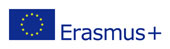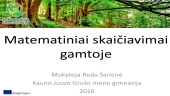This project has been funded with support from the European Commission.
This web site reflects the views only of the author, and the Commission cannot be held responsible for any use which may be made of the information contained therein.

Also available in: fr el ie it lt pt ro en fb yt

# E-lessons

Homepage > Learning Science through Nature > E-lessons

E-LESSON DATATITLE OF THE E-LESSON
Mathematical calculations in the wild
COUNTRY
Lithuania
INTRODUCTORY TEXT TO THE LESSON
- The contents of the lesson:
The students were divided into five groups (2-3 students in a group), they were given envelopes with the tasks inside. The students read the tasks aloud. They were given 30 minutes for task completion.
The lesson took place in the reserve of Dubrava urotshistshe. The first group of students had to calculate the beam of the highest pine (They used only a roulette). The second group calculated the height in which the tree had broken (the feature of similar triangles). The third group had to calculate the length of the survey path in meters using a pedometer and to calculate what percentage of the whole path includes the path through the marsh. The students of the fourth group had to convert the length of the survey path into ancient units of measurement. The fifth group had to draw a natural object and calculate the number of times it became bigger after it had been transferred onto a sheet of paper. After having completed the tasks, the students had to present them and summarize.
- The learning objective: To perform mathematical calculations by using the objects existing in the wild.
THEMATIC AREA
Math
TARGET AGE GROUP
17
VIDEO